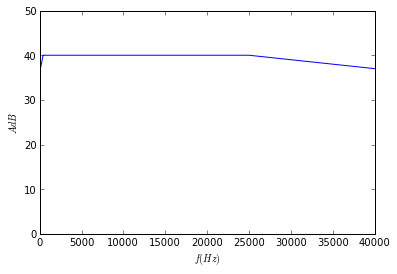# Chapter 9:Multi stage Amplifiers¶

### Example 9.1 Page no.305¶

In :
#Given
A1=30                         #voltage gain 1
A2=50                        #voltage gain  2
A3=80                        #voltage gain  3

#Calculation
import math
A=A1*A2*A3             #overall Voltage Gain
# Result
print " The overall Voltage Gain of the Multistage Amplifier Adb =  ",round(Adb,2),"dB"

 The overall Voltage Gain of the Multistage Amplifier Adb =   101.58 dB


### Example 9.2 Page no.312¶

In :
Vcc=30.0                                    #V, collector bias junction voltage
Vi=1.4                                      #V, input voltage
Vbe=0.7                                  #V. base emitter voltage
B=300                                     #Beeta, gain factor
R1=27000.0                            #Ohms, given resistance
R2=680.0                                #Ohms given resistance
R3=24000.0                            #Ohms
R4=2400.0                              #Ohms

#Calculation
Ve=Vi-Vbe                                            #V, voltage at emitter terminal
Ie1=Vbe/R2                                          #A, emitter current at 1st stage
Ic1=Ie1                                                 #A, collector current
Vc1=Vcc-round(Ie1,3)*R1                                #collector voltage at 1st stage
Vb2=Vc1                                             #V, base voltage at 2nd stage

Ve2=Vb2-Vbe                                      #V  emitter voltage at 2nd stage
Ie2=Ve2/R4                                         #A,  emitter current at 2nd stage
Ic2=round(Ie2,3)                                               #A  collector  current at 2nd stage
Vc2=Vcc-Ic2*R3
Vo=Vc2
#Displaying The Results in Command Window
print " The Voltage at the Output Terminal of Two Stage Direct Coupled Amplifier, Vo = ",Vo,"V"

 The Voltage at the Output Terminal of Two Stage Direct Coupled Amplifier, Vo =  6.0 V


### Example 9.3 Page no.319¶

In :
A=100                         #voltage gain
f1=400                        #Hz, frequency 1
f2=25*10**3                   #Hz, frequency  2
f3=80                         #Hz, frequency 3
f4=40*10**3                   # Hz, frequency 4

#Calculation
import math
# Result
print " The Gain at Cutoff Frequencies is, Adb (at Cutoff Frequencies) =  ",Adbc,"dB"

#plot
from pylab import *
f1=[80,400,25000,40000]
xlim(0,40000)
xlabel("$f(Hz)$")
ylabel("$AdB$")
ylim(0,50)
show(a1)

 The Gain at Cutoff Frequencies is, Adb (at Cutoff Frequencies) =   37.0 dB### Example 9.4 Page no 325.¶

In :
                                #all the quantities of R are resistances
R1=5600.0                                       #Ohms
R2=56000.0                                     #Ohms
R3=1100.0                                       #Ohms

#Calculation
Zi=R1*R2*R3/(R1*R2+R2*R3+R3*R1)
#Result
print " The Input Impedance, Zi =   ",round(Zi/10**3,3),"kohm"

#(b) Calculate output Impedance
Ro1=3300.0                                   #Ohms
Ro2=2200                                #Ohms

#Calculation
Zo=Ro1*Ro2/(Ro1+Ro2)

#Result
print " The Output Impedance, Zo =  ",Zo/10**3,"kohm"
#(c) voltage gain
hfe=120                                        #current amplification factor
hie=1100.0                                    #Ohms, dynamic input resistance
R1=6800.0                                    #Ohms
R2=56000.0                                  #Ohms
R3=5600.0                                    #Ohms
R4=1100.0                                    #Ohms

#Calculation
Rac2=Ro1*Ro2/(Ro1+Ro2)
A2=-hfe*Rac2/hie
Rac1=R1*R2*R3*R4/(R1*R2*R3+R2*R3*R4+R1*R3*R4+R1*R2*R4)
Rac1=round(Rac1,0)
A1=-hfe*Rac1/hie

A1=round(A1,2)
A=A1*A2                                 #Overall Gain

#Result
print " The Overall Gain, A = ",round(A,0)

 The Input Impedance, Zi =    0.905 kohm
The Output Impedance, Zo =   1.32 kohm
The Overall Gain, A =  12535.0


### Example 9.5 Page no. 326¶

In :
Rl=10000.0                                          #Ohms, resistance
Rg=470000.0                                      #Ohms dynamic input resistance
Cs=100*10**(-12)                             #F Capacitance
u=25                                                     #amplification factor
rp=8000.0                                          #Ohms
Cc=0.01*10**(-6)                             #F, capacitance

#Calculation
import math
gm=u/rp                                           #transconductance
Req=rp*Rl*Rg/(rp*Rl+Rl*Rg+Rg*rp)                 #equivalent resistance
Avm=(u/rp)*Req                                  #voltage gain
Avmd=Avm**2                               # Voltage Gain of Two Stages
Rd=(rp*Rl/(rp+Rl))+Rg
f1=1/(2*math.pi*Cc*Rd)                     #Lower Cutoff Frequency
f1d=f1/math.sqrt(math.sqrt(2)-1)                    #Lower Cutoff Frequency of Two Stages
Req =(rp*Rl)/(rp+Rl) #approximately
f2=1/(2*math.pi*Cs*Req)                  #Upper Cutoff Frequency
f2d=f2*math.sqrt(math.sqrt(2)-1)                   #Upper Cutoff Frequency of Two Stages
BW=f2d-f1d
#Bandwidth
# Result
print " The Voltage Gain of Two Stages, Avmd =  ",round(Avmd,2)
print " The Bandwidth, BW = ",round(BW/10**3,0),"KHz"

 The Voltage Gain of Two Stages, Avmd =   189.3
The Bandwidth, BW =  230.0 KHz